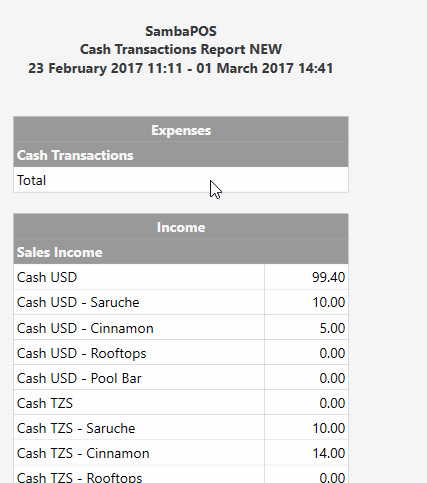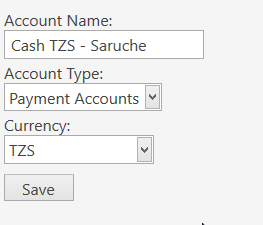# Cash transaction report to show foreign currency amounts

How do I get amounts in the Cash Transaction Report to show using the currency for the account, e.g. the TZS accounts are using TZS currency but instead get shown as the USD amount based on the exchange rate setup in SambaPOSExample part from report:

``````[Income:1,2, 1]
>Sales Income||
Cash USD||{ACCOUNT TRANSACTION TOTAL:Payment Transaction:Cash}
Cash USD - Saruche||{ACCOUNT TRANSACTION TOTAL:Payment Transaction:Cash - Saruche}
Cash USD - Cinnamon||{ACCOUNT TRANSACTION TOTAL:Payment Transaction:Cash - Cinnamon}
Cash USD - Rooftops||{ACCOUNT TRANSACTION TOTAL:Payment Transaction:Cash - Rooftops}
Cash USD - Pool Bar||{ACCOUNT TRANSACTION TOTAL:Payment Transaction:Cash - Pool Bar}
Cash TZS||{ACCOUNT TRANSACTION TOTAL:Payment Transaction:Cash TZS}
Cash TZS - Saruche||{ACCOUNT TRANSACTION TOTAL:Payment Transaction:Cash TZS - Saruche}
Cash TZS - Cinnamon||{ACCOUNT TRANSACTION TOTAL:Payment Transaction:Cash TZS - Cinnamon}
Cash TZS - Rooftops||{ACCOUNT TRANSACTION TOTAL:Payment Transaction:Cash TZS - Rooftops}
Cash TZS - Pool Bar||{ACCOUNT TRANSACTION TOTAL:Payment Transaction:Cash TZS - Pool Bar}
...
``````I would guess that you will need to use an expression to convert it. At least, that is what I needed to do with my Cashout Count report. And it is even worse:

Report Tags seem to give values in Default currency. And Reports have no access to the `{EXCHANGE RATE:X}` tag either. So in my case, I need to store the XR in a Program Setting like `{SETTING:XR_USDtoHNL}`, then do this:

``````[=F(TN('{ACCOUNT TOTAL:Cash HNL}')*TN('{SETTING:XR_USDtoHNL}'),'0.00')]
``````

Without that ^ conversion, the Cash HNL Account Total would be shown in Default (USD). This might be something that @emre could “fix”but it might not be desirable in many cases, so I am fine with the way it is now, save for the fact it might be nice to be able to access the `{EXCHANGE RATE:X}` tag in Reports - that would help.

Here is that full Report - you can see the conversions all over the place:

``````[Cashout Report:1, 1, 1, 1, 1]
>>Tender|Count|(-Float)|Account|+/-
HNL|[=F(TN('{SETTING:HNLcountTotal}'),'0.00')]|[=F(TN('{SETTING:HNLcountTotal}')-TN('{SETTING:HNLfloat}'),'0.00')]|[=F(TN('{ACCOUNT TOTAL:Cash HNL}')*TN('{SETTING:XR_USDtoHNL}'),'0.00')]|[=F(TN('{SETTING:HNLcountTotal}')-TN('{SETTING:HNLfloat}')-(TN('{ACCOUNT TOTAL:Cash HNL}')*TN('{SETTING:XR_USDtoHNL}')),'0.00')]
USD|[=F(TN('{SETTING:USDcountTotal}'),'0.00')]|[=F(TN('{SETTING:USDcountTotal}')-TN('{SETTING:USDfloat}'),'0.00')]|[=F(TN('{ACCOUNT TOTAL:Cash USD}'),'0.00')]|[=F(TN('{SETTING:USDcountTotal}')-TN('{SETTING:USDfloat}')-TN('{ACCOUNT TOTAL:Cash USD}'),'0.00')]
CC HNL|[=F(TN('{SETTING:CCcountTotal}'),'0.00')]|-|[=F(TN('{ACCOUNT TOTAL:Credit Card HNL}'),'0.00')]|[=F(TN('{SETTING:CCcountTotal}')-TN('{ACCOUNT TOTAL:Credit Card HNL}'),'0.00')]
CC USD|[=F(TN('{SETTING:CCUSDcountTotal}'),'0.00')]|-|[=F(TN('{ACCOUNT TOTAL:Credit Card USD}'),'0.00')]|[=F(TN('{SETTING:CCUSDcountTotal}')-TN('{ACCOUNT TOTAL:Credit Card USD}'),'0.00')]
>>Account|HNL|USD|-|TTL USD
Tips HNL|[=F(TN('{ACCOUNT TOTAL:Tips}')*TN('{SETTING:XR_USDtoHNL}'))]|[=F(TN('{ACCOUNT TOTAL:Tips}'))]|-|[=F(TN('{ACCOUNT TOTAL:Tips}'))]
Round|[=F(TN('{ACCOUNT TOTAL:Rounding HNL}'))]|[=F(TN('{ACCOUNT TOTAL:Rounding USD}'))]|-|[=F(TN('{ACCOUNT TOTAL:Rounding USD}')+TN('{ACCOUNT TOTAL:Rounding HNL}'))]
>XR/TTL|[=F(TN('{SETTING:XR_USDtoHNL}'),'0.00')]|[=F(TN('{SETTING:XR_HNLtoUSD}'),'0.000000')]|-|[=F(TN('{ACCOUNT TOTAL:Tips}')+TN('{ACCOUNT TOTAL:Rounding USD}')+TN('{ACCOUNT TOTAL:Rounding HNL}'))]

>>TOTALS|Count|(-Float)|Account|+/-
HNL|[=F(TN('{SETTING:HNLcountTotal}')+TN('{SETTING:CCcountTotal}')+TN('{SETTING:CCUSDcountTotal}')*TN('{SETTING:XR_USDtoHNL}')+TN('{SETTING:USDcountTotal}')*TN('{SETTING:XR_USDtoHNL}'),'0.00')]|[=F( TN('{SETTING:HNLcountTotal}')-TN('{SETTING:HNLfloat}') + TN('{SETTING:USDcountTotal}')*TN('{SETTING:XR_USDtoHNL}')-TN('{SETTING:USDfloat}')*TN('{SETTING:XR_USDtoHNL}') + TN('{SETTING:CCcountTotal}') + TN('{SETTING:CCUSDcountTotal}')*TN('{SETTING:XR_USDtoHNL}'),'0.00')]|[=F((TN('{ACCOUNT TOTAL:Cash HNL}')+TN('{ACCOUNT TOTAL:Cash USD}')+TN('{ACCOUNT TOTAL:Credit Card HNL}')+TN('{ACCOUNT TOTAL:Credit Card USD}')+TN('{ACCOUNT TOTAL:Tips}'))*TN('{SETTING:XR_USDtoHNL}'),'0.00')]|[=F(TN('{SETTING:HNLcountTotal}')-TN('{SETTING:HNLfloat}') + TN('{SETTING:USDcountTotal}')*TN('{SETTING:XR_USDtoHNL}')-TN('{SETTING:USDfloat}')*TN('{SETTING:XR_USDtoHNL}') + TN('{SETTING:CCcountTotal}') + TN('{SETTING:CCUSDcountTotal}')*TN('{SETTING:XR_USDtoHNL}') - ((TN('{ACCOUNT TOTAL:Cash HNL}')+TN('{ACCOUNT TOTAL:Cash USD}')+TN('{ACCOUNT TOTAL:Credit Card HNL}')+TN('{ACCOUNT TOTAL:Credit Card USD}')+TN('{ACCOUNT TOTAL:Tips}')))*TN('{SETTING:XR_USDtoHNL}'),'0.00')]
USD|[=F(((TN('{SETTING:HNLcountTotal}')+TN('{SETTING:CCcountTotal}'))/TN('{SETTING:XR_USDtoHNL}'))+TN('{SETTING:CCUSDcountTotal}')+TN('{SETTING:USDcountTotal}'),'0.00')]|[=F( ((TN('{SETTING:HNLcountTotal}')-TN('{SETTING:HNLfloat}'))/TN('{SETTING:XR_USDtoHNL}')) + TN('{SETTING:USDcountTotal}')-TN('{SETTING:USDfloat}') + (TN('{SETTING:CCcountTotal}')/TN('{SETTING:XR_USDtoHNL}')) + TN('{SETTING:CCUSDcountTotal}'),'0.00')]|[=F(TN('{ACCOUNT TOTAL:Cash HNL}')+TN('{ACCOUNT TOTAL:Cash USD}')+TN('{ACCOUNT TOTAL:Credit Card HNL}')+TN('{ACCOUNT TOTAL:Credit Card USD}')+TN('{ACCOUNT TOTAL:Tips}'),'0.00')]|[=F(TN('{SETTING:HNLcountTotal}')/TN('{SETTING:XR_USDtoHNL}')-TN('{SETTING:HNLfloat}')/TN('{SETTING:XR_USDtoHNL}') + TN('{SETTING:USDcountTotal}')-TN('{SETTING:USDfloat}') + TN('{SETTING:CCcountTotal}')/TN('{SETTING:XR_USDtoHNL}') + TN('{SETTING:CCUSDcountTotal}') - (TN('{ACCOUNT TOTAL:Cash HNL}')+TN('{ACCOUNT TOTAL:Cash USD}')+TN('{ACCOUNT TOTAL:Credit Card HNL}')+TN('{ACCOUNT TOTAL:Credit Card USD}')+TN('{ACCOUNT TOTAL:Tips}')),'0.00')]
``````
1 Like

Along the lines of this topic… I am hoping to use SambaPOS to store the vaues of money that I have in my till and safe over time, and not just view the monetary movements of just one work period. For example, this is that I would like to have for my Till (USD) and Safe (USD) reports at the end of each day…

``````Till (USD)
Start of day           235.00
Move from safe       +1000.00
Sales                 +125.00
Expenses              -985.00
Refunds                -25.00
End of day             350.00

Safe (USD)
Start of day         3540.00
Move from till         +0.00
Move to till        -1000.00
End of day           2540.00
``````

Using the `{ACCOUNT BALANCE:XXX}` tag I am able to find this end of day figure which gives the actual value rather than `{ACCOUNT TOTAL:XXX}` which only give a work period total.

The problem I have is when it comes to getting my hands on the foreign currency figure using `{ACCOUNT BALANCE:XXX}`. As @QMcKay says, it is returned in the native currency. Although his method of pulling the currency exchange rate works well if you are looking only at work period transactions, it does not work for historic values outside of the work period since the rate will have changed over time. However, the accounts screen does give me the correct values. So, how can I get access to the values from the accounts screen for use in a custom report?

Have you missed safe --> bank/back pocket?Safe --> Bank… Definitely Bank, nothing else. Scandalous comments there @JTRTech!

Yes, I have other transactions like that in there too, but I left them out for the sake of brevity.

This is similar to the problem I had for reporting End of Day Cashouts. I had been using a Program Setting, but that was only good for 1 day. So I turned everything into Tasks, which allows for historical tracking via `{REPORT TASK DETAILS:X}`.

The same was true of my Exchange Rate, which affects Cashouts, so I also needed to store it as a Task. Now I can see what the Rate was for any given day from the past.

Now my Cashout Report looks like this, so if I change the Date in the Report Explorer, the correct Rate for that Day is used for calculations:

``````[Cashout Count Report:1, 1, 1, 1, 1]

>>CASH|Count|(-Float)|Account|+/-

>>CC|Count|(+Devo)|Account|+/-
CC USD|[=F(TN('{REPORT TASK DETAILS:TSC.Total.Sum:(TST=CO Card USD)}'),'0.00')]|-|[=F(TN('{ACCOUNT TOTAL:Credit Card USD}'),'0.00')]|[=F(TN('{REPORT TASK DETAILS:TSC.Total.Sum:(TST=CO Card USD)}')-TN('{ACCOUNT TOTAL:Credit Card USD}'),'0.00')]

>>CC Tips|Count|Paid|Account|+/-

>>Account|HNL|USD|-|TTL USD
Round|[=F(TN('{ACCOUNT TOTAL:Rounding HNL}'))]|[=F(TN('{ACCOUNT TOTAL:Rounding USD}'))]|-|[=F(TN('{ACCOUNT TOTAL:Rounding USD}')+TN('{ACCOUNT TOTAL:Rounding HNL}'))]

>>TOTALS|Count|(-Float)|Account|+/-
``````

I get the advantage of sotring the monetary totals day-to-day in tasks to allow you to go back in time and see what you had, but it does seem strange that you have to do this to calculate the running balance when it is available right there in the accounts screen. Do you think I should raise a feature request, or do you think this task-based approach is going to be the only way to do this?

The Account Screens are really rather primitive, and are simply showing results of simple SQL Queries. The Accounts Screens don’t actually know what the Exchange Rate is. They are just showing the values from the `[AccountTransactionValues]` table in the DB, namely `[Debit]`, `[Credit]`, and `[Exchange]` (converted Foreign Currency Amount) for each Account. When it sees that Debit/Credit does not match Exchange, it converts the Debit/Credit fields to the Account Currency by calculating the XR for that Transaction. You could derive the XR from that using Reports in the same way, but the XR itself is not stored in the Account tables.

It might be useful (simpler) to store the XR in the record as well, but so far this is not the case, and it’s probably not a priority request after all this time.

Just trying to write my own SQL to squeeze in here, but I’m scratching my head at the fact there is an `AccountTransactions` and an `AccountTransactionValues` table which contains much of the same information.

What is the difference between the two? Which set of data should I work with to write the script?

Using `AccountTransactionValues` seems to work with this script, am I missng anything silly?..

``````SELECT        SUM(ROUND(AccountTransactionValues.Exchange, 2)) AS Balance
FROM            AccountTransactionValues INNER JOIN
Accounts ON AccountTransactionValues.AccountId = Accounts.Id
WHERE        (Accounts.Name = 'Cash (MXN)')
``````

`[AccountTransactions]` is more of a summary in that it contains less granular information. In general, I never use that Table, because it doesn’t give me enough detail, ,mostly because there is only reference to Transaction Type, and Account Types, with no detail as to the Source and Target Accounts themselves. But it keeps a Transaction down to 1 line.

`[AccountTransactionValues]` is more detailed in that it directly references the Account for the Transaction. There should be matching Debit and Credit records (2 records per Transaction) so that you can determine the Source and Target Accounts for the Transaction.

Your query looks fine to me.

Here is one that gives very good detail - I use this as my basis quite a bit and trim it down to focus on just the data I want for the moment:

``````SELECT
tv.[Id]
,tv.[Date]
,[Debit]
,[Credit]
,[Exchange]
,CASE WHEN [Exchange]<0 THEN -1 * ROUND(1 / ( ([Debit]+[Credit]) / [Exchange] ),2)
ELSE ROUND(1 / ( ([Debit]+[Credit]) / [Exchange] ),2)
END as [XR]
,isnull(fc.[Name],'USD') as [Currency]
--,tv.[AccountTypeId]
--,acctyp.[Name] as [accType]
--,[AccountId]
,a.[Name] as [Account]
,tv.[Name]
--,[AccountTransactionTypeId]
,tt.[Name] as [txType]
--,[AccountTransactionId]
,acctx.[Name] as [txName]
,dt.[Name] as [docType]
--,[AccountTransactionDocumentId]
,d.[Name] as [docName]
--,[DepartmentId]

FROM [AccountTransactionValues] tv
LEFT JOIN [Accounts] a on a.[Id] = tv.[AccountId]
LEFT JOIN [AccountTypes] acctyp on acctyp.[Id] = tv.[AccountTypeId]
LEFT JOIN [AccountTransactions] acctx on acctx.[Id] = tv.[AccountTransactionId]
LEFT JOIN [AccountTransactionTypes] tt on tt.[Id] = tv.[AccountTransactionTypeId]
LEFT JOIN [AccountTransactionDocuments] d on d.[Id] = tv.[AccountTransactionDocumentId]
LEFT JOIN [AccountTransactionDocumentTypes] dt on dt.[Id] = d.[DocumentTypeId]
LEFT JOIN [ForeignCurrencies] fc on fc.[Id] = a.[ForeignCurrencyId]

WHERE 1=1
AND tv.[Date] >= '2017-05-01'
AND a.[Name] LIKE '%Cash%HNL%'
``````

Very good detail:

help me I don can customize report income currency I see way above I don understand
I cash USD = 9 USD
and cash LAK = 3000 LAK
but Output USD = 72000 LAK
I want output USD = 9 USD is not 72000 LAK

I try
[=F(TN(’{ACCOUNT TOTAL:LAK}’)*TN(’{SETTING:XR_LAKtoUSD}’),‘0.00’)]
it not work

If you just want the value of the USD account then you just need the following:

1 Like

Or do what you did with LAK

{ ACCOUNT TRANSACTION TOTAL:Payment Transaction:USD }

1 Like

help me how can i do? @RickH

not work @RickH i set default is LAK
i want out output { ACCOUNT TRANSACTION TOTAL:Payment Transaction:USD } / Rete USD
how can i do?

I have been doing a lot of work on this recently, but I ran in to issues when reading the exchange rate from a setting, becaue this is only reading today’s exchange rate. If I am looking at historical report the output produced is of no use.

To get around this I use this report:

``````[Daily totals:2, 1, 1]
>Type|MXN|USD
Till USD| |[=F(TN('{ACCOUNT TOTAL:Till USD}'))]
Till MXN|[=F(TN('{REPORT SQL DETAILS:SELECT SUM(ROUND(Exchange, 2)) as [Total] FROM AccountTransactionValues where AccountId = 10 and [Date] > '{Start}' and [Date] < '{End}':F.Total}'))]|
``````

For me, account 10 is my “Tll MXN” account, so it shows me what I should have in the till right now (and what I would have had in the past when looking at historic reports.

You can also see what you recived as sales for a given period using this report:

``````[Sales totals:2, 1, 1]
>Type|Quantity|Total
Cash USD|{REPORT PAYMENT COUNT:(PT=SALES Cash USD)}|[=F(TN('{REPORT SQL DETAILS:SELECT SUM(ROUND(Exchange, 2)) AS [Total] FROM AccountTransactionValues where AccountId = 5 and (AccountTransactionTypeId = 4 or AccountTransactionTypeId = 11) and [Date] > '{Start}' and [Date] < '{End}':F.Total}'))]
Cash MXN|{REPORT PAYMENT COUNT:(PT=SALES Cash MXN)}|[=F(TN('{REPORT SQL DETAILS:SELECT SUM(ROUND(Exchange, 2)) as [Total] FROM AccountTransactionValues where AccountId = 10 and (AccountTransactionTypeId = 4 or AccountTransactionTypeId = 12) and [Date] > '{Start}' and [Date] < '{End}':F.Total}'))]
``````

These reports include only the sales and change transactions for a particular account (Till USD or Till MXN). This way you can see the true value of the sales on any day, before any other cash movements (perhaps expenses or you moving large amounts of cash from the till to the safe for security).

2 Likes

@QMcKay I’m stuck with using the task type Exchange Rate as you mentioned above. Are there any actions or rules that are needed for it to work?

In my case we accept payment in SRD, USD and Euro. Our main currency is USD so the cash transaction report shows all the account values in USD. I need to display euro cash accounts in euro so the staff can count their cash easier.